# Frustum of a cone

A reservoir contains 28.54 m3 of water when completely full. The diameter of the upper base is 3.5 m while at the lower base is 2.5 m. Determine the height if the reservoir is in the form of a frustum of a right circular cone.

Correct result:

h =  4.0005 m

#### Solution: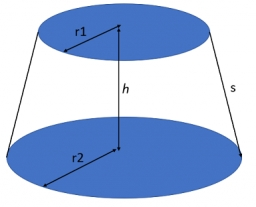We would be pleased if you find an error in the word problem, spelling mistakes, or inaccuracies and send it to us. Thank you!Tips to related online calculators
Looking for a statistical calculator?
Tip: Our volume units converter will help you with the conversion of volume units.

## Next similar math problems:

• A concrete pedestalA concrete pedestal has a shape of a right circular cone having a height of 2.5 feet. The diameter of the upper and lower bases are 3 feet and 5 feet, respectively. Determine the lateral surface area, total surface area, and the volume of the pedestal.
• Heptagonal pyramidA hardwood for a column is in the form of a frustum of a regular heptagonal pyramid. The lower base edge is 18 cm and the upper base of 14 cm. The altitude is 30 cm. Determine the weight in kg if the density of the wood is 10 grams/cm3.
• Two vases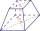Michaela has two vases in her collection. The first vase has the shape of a cone with a base diameter d = 20 cm; the second vase has the shape of a truncated cone with the diameter of the lower base d1 = 25 cm and with the diameter of the upper base d2 =
• The diagram 2The diagram shows a cone with slant height 10.5cm. If the curved surface area of the cone is 115.5 cm2. Calculate correct to 3 significant figures: *Base Radius *Height *Volume of the cone
• Volume and surfaceCalculate the volume and surface area of the cylinder when the cylinder height and base diameter is in a ratio of 3:4 and the area of the cylinder jacket is 24 dm2.
• Truncated cone 3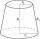The surface of the truncated rotating cone S = 7697 meters square, the substructure diameter is 56m and 42m, determine the height of the tang.
• Angle of coneThe cone has a base diameter of 1.5 m. The angle at the main apex of the axial section is 86°. Calculate the volume of the cone.
• Lateral surface areaThe ratio of the area of the base of the rotary cone to its lateral surface area is 3: 5. Calculate the surface and volume of the cone, if its height v = 4 cm.
• Digging a pit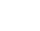The pit has the shape of a regular quadrilateral truncated pyramid. The edges of the bases are 14m and 10m long. The sidewalls form an angle of 135° with a smaller base. Determine how many m3 of soil were excavated when digging the pit?
• Water reservoir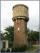The cuboid reservoir contains 1900 hectoliters of water and the water height is 2.5 m. Determine the dimensions of the bottom where one dimension is 3.2 m longer than the second one.
• Spherical segmentCalculate the volume of a spherical segment 18 cm high. The diameter of the lower base is 80 cm, the upper base 60 cm.
• Truncated cone 5The height of a cone 7 cm and the length of side is 10 cm and the lower radius is 3cm. What could the possible answer for the upper radius of truncated cone?
• Base of prismThe base of the perpendicular prism is a rectangular triangle whose legs length are at a 3: 4 ratio. The height of the prism is 2cm smaller than the larger base leg. Determine the volume of the prism if its surface is 468 cm2.
• Truncated coneCalculate the height of the rotating truncated cone with volume V = 1115 cm3 and a base radii r1 = 7.9 cm and r2 = 9.7 cm.
• PrismThe volume of tetrahedral prism is 2.43 m3. Base of prism is a parallelogram in which a side 2,5dm and height ha = 18cm. Calculate the height of the prism.
• Slant heightThe slant height of cone is 5cm and the radius of its base is 3cm, find the volume of the cone
• Conical bottleWhen a conical bottle rests on its flat base, the water in the bottle is 8 cm from it vertex. When the same conical bottle is turned upside down, the water level is 2 cm from its base. What is the height of the bottle?